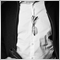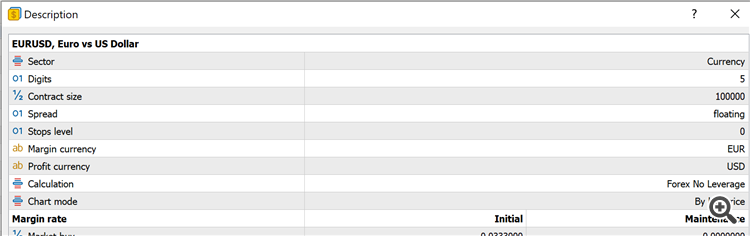# Symbol Point Value - page 2178

Fernando Carreiro #:

Here are two examples from AMP Global (Europe):

• Micro E-mini S&P 500 (Futures): point size = 0.01, tick size = 0.25, tick value = \$1.25
• EURO STOXX Banks (Stock Index): point size = 0.01, tick size = 0.05, tick value = €2.50
I’ll set up a demo account and try it out. Thanks.178

EdFuk #:
I’ll set up a demo account and try it out. Thanks.

I've opened up an account and in testing my new code I can see that the DeltaValuePerLot function is not returning in my account currency.  A stop loss was hit and the value was approx 1.318 smaller than it should have been which is the GBPUSD exchange rate at the moment.

It looks like I will need to check what currency the following returns. Any ideas how I go about that?

`SymbolInfoDouble(pair, SYMBOL_TRADE_TICK_VALUE)`2335

I am not sure, but I would expect this to be in the profit currency of the symbol, if it's not in the account currency.

I think I have read on this forum, brokers set this value wrongly, sometimes. Therefore it seems not reliable across all brokers.

I for my usage have written a function to calculate this value for me, not relying on the API in this matter.178

Hi Everyone,

On testing my new code which now calculates position size using tick value (thanks Fernando) I had the same experience as some previous posters regarding what currency is returned when the tick value is retrieved using the SymbolInfoDouble(CurrentSymbol,SYMBOL_TRADE_TICK_VALUE). After trying this out myself I have found that the currency is dependent on the symbol and also the broker.

For example, I compared the output using EURUSD and also SPX500 for my account which is in GBP. Going onto the website the pip value for SPX500 is 1USD. Retrieving the value in MQL5 gives a value of 1 which is obviously 1USD – not in my account currency.

For EURUSD the pip value given on the website is 10USD therefore the point value is 1USD per point. 1 point is equal to 1 tick in this case the tick value should be 1. Retrieving the value in MQL5 I get a value of 0.7599 which is the value in GBP, using the exchange rate at the time.

It is crucial to know what currency the tick value is given in (by the broker) in order to calculate position sizes and monetary values etc correctly so I’ve been trying to find where this information is given within MT5/MQL5. Unfortunately I have struggled and cannot find where to get this information. From what I can see this information is not even provided by the broker themselves on their website but maybe I’m just not looking in the right place.

Where can I find this information so that I can develop robust code to handle all situations?

Cheers,

Ed178

Dominik Christian Egert #:
I am not sure, but I would expect this to be in the profit currency of the symbol, if it's not in the account currency.

I think I have read on this forum, brokers set this value wrongly, sometimes. Therefore it seems not reliable across all brokers.

I for my usage have written a function to calculate this value for me, not relying on the API in this matter

Dominik, yes this is exactly what I have found. How are you calculating the value? It seems cray that there is no way of knowing what currency the tick_value is given in. It's so crucial.

Cheers,

Ed2335

Well, I construct the symbol for finding the quote between account currency and profit currency of the symbol in question.

Then I call my FindSymbol function which returns me the symbol and then I get the current quote. Then I calculate the value of one tick from my Chartsymbol as account currency value.

It can happen, you need to utilize 2 hidden symbols.

I figured, it is much more reliable to use the base/profit/margin currency details from symbols to identify the symbols names correctly, since the brokers symbol names are more or less arbitrary. (For the symbol search function)

The rest is simple math, converting between the currency pairs.

```        const bool mm_calc_margin_currency(const string margin_currency, const string profit_currency, string& calculation_currency, bool& rev_quote)
{
// Local init

bool    custom_symbol           = false;
string  symbolname              = NULL;
string  m_cur                   = NULL;
string  p_cur                   = NULL;

// Initially try guess a symbol

if( (SymbolExist(margin_currency + profit_currency, custom_symbol))
&& (!custom_symbol))
{ calculation_currency = margin_currency + profit_currency; rev_quote = true; return(true); }

if( (SymbolExist(profit_currency + margin_currency, custom_symbol))
&& (!custom_symbol))
{ calculation_currency = profit_currency + margin_currency; rev_quote = false; return(true); }

// Search all available symbols for a match

for(int cnt = 0; (cnt < SymbolsTotal(false)) && !IsStopped(); cnt++)
{
// Get symbol name
symbolname = SymbolName(cnt, false);

// Get margin currency
m_cur = SymbolInfoString(symbolname, SYMBOL_CURRENCY_MARGIN);

// Get profit currency (profit on price change)
p_cur = SymbolInfoString(symbolname, SYMBOL_CURRENCY_PROFIT);

// Check if found
if(      ( (m_cur == margin_currency)
&& (p_cur == profit_currency))
||      ( (p_cur == margin_currency)
&& (m_cur == profit_currency)) )
{
calculation_currency = symbolname;
if(SymbolExist(calculation_currency, custom_symbol) && (!custom_symbol))
{
rev_quote = (m_cur == margin_currency);
return(true);
}
}
}

// Return failure
return(false);
}

double MathRange(const double value, const double min, const double max)
{ return((value * ((value >= min) && (value <= max))) + (min * (value < min)) + (max * (value > max))); }```

Here as a start on searching for symbols, a function that I have posted once on the forum.

It's meant to be an example for a search function. My current version has changed since then, but I guess you get the idea.178

Dominik Christian Egert #:
Well, I construct the symbol for finding the quote between account currency and profit currency of the symbol in question.

Then I call my FindSymbol function which returns me the symbol and then I get the current quote. Then I calculate the value of one tick from my Chartsymbol as account currency value.

It can happen, you need to utilize 2 hidden symbols.

I figured, it is much more reliable to use the base/profit/margin currency details from symbols to identify the symbols names correctly, since the brokers symbol names are more or less arbitrary. (For the symbol search function)

The rest is simple math, converting between the currency pairs.

Here as a start on searching for symbols, a function that I have posted once on the forum.

It's meant to be an example for a search function. My current version has changed since then, but I guess you get the idea.

Thanks Dominik. I guess the big question is how do you know if the tick value currency is in your account currency or another one? That is the big question here.

Cheers,

Ed2335

I don't. I just skip the question and compute it myself always.178

Dominik Christian Egert #:
I don't. I just skip the question and compute it myself always.

Hmm OK. Sorry I'm struggling to get my head around this. So, let's look at an example. Case 1 - EURUSD and Case 2 - SPX500. The specification from given by my broker is as shown below:

CASE 1The tick value for Case 1 - EURUSD is given in my Account Currency GBP wheras for Case 2- SPX500 it's given in USD.

So if you're bypassing the tick value data given by the broker how do you actually calculate it? Are you using the tick size provided by the broker?

Seems crazy that this is not rigorously set up by the brokers. Does the calculation type (Forex No leverage and CFD in these cases) hold clues to the way tick value is specified?

Apologies for being a numpty on this one and thanks for the help.2335

As I said, I >ignore< this specific value.

I still calculate the tick value in account currency myself. I use quotes of currencies to do that.

In your case, for SPX it's Profit-Currency to account currency.

You take the symbol GBPUSD and get the quote. Then you calculate the account value from it.

Same goes for example 1. You get the quote of GBPUSD.

Edit: you need only the current prices. That's all. Tick size comes after you have the tick value.